Landmark SLAM Using AprilTag Markers

This example shows how to combine robot odometry data and observed fiducial markers called AprilTags to better estimate the robot trajectory and the landmark positions in the environment.

Download the .mat file that contains the raw data recorded from a rosbag on a ClearPath Robotics™ Jackal™. The raw data includes:

• Images taken using an Axis™ M1013 network camera at the resolution of 640x480.

• Odometry data pre-processed and synchronized with the image data.

The rosbag is imported into MATLAB™ and stored as a .mat file. To see how the data is extracted from the rosbag and pre-processed, the exampleHelperExtractDataFromRosbag example helper is provided at the end of this example. ROS Toolbox is required to use the helper function.

unzip(filename);

Build Pose Graph From Sensor Data

In this example, a set of AprilTag markers are printed and randomly placed in the test environment. The tags are treated as landmarks in the pose graph. Given the camera intrinsics and the size of the tag, you can convert the images with AprilTag observations into point landmark measurements using the readAprilTag (Computer Vision Toolbox) function. These landmark measurements are relative to the current robot frame. To find out the intrinsic parameters of your camera, follow the steps in the Camera Calibration Using AprilTag Markers (Computer Vision Toolbox) example or use a checkerboard pattern with the Camera Calibrator (Computer Vision Toolbox) app. The AprilTag markers used in this example are taken from the "tag46h11" family with no duplicate IDs. The tag size printed is 136.65 mm.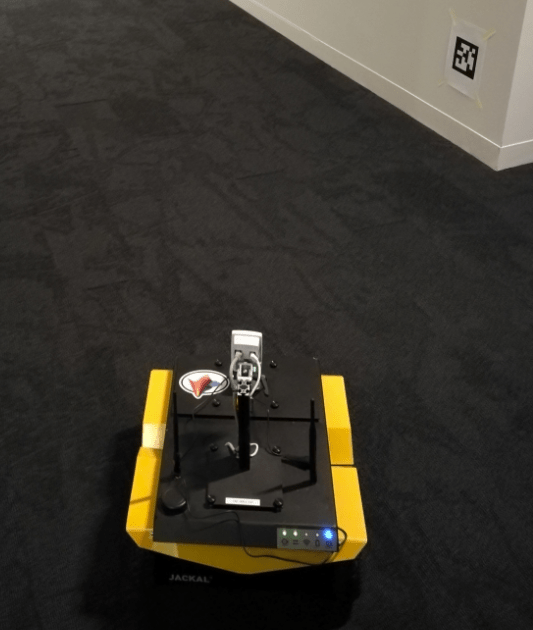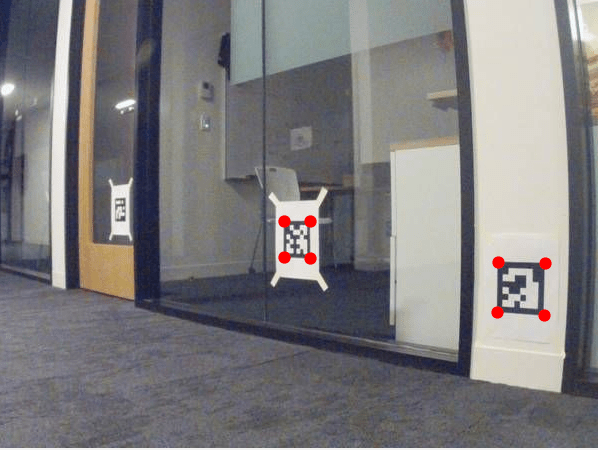tagFamily = "tag36h11";
tagSize = 136.65; % mm

After importing the synchronized sensor data and the camera parameters, build a pose graph of node estimates from the measurements in the sensor data. The pose graph contains node estimates, edge contraints, and loop closures that estimate robot poses.

% Create a pose graph object
pg = poseGraph3D;

By using fiducial markers like AprilTags, the block pattern in the image provides unique IDs for each landmark observation. This ID information reduces the need for difficult data association algorithms when performing simultaneous localization and mapping (SLAM).

Create the poseGraph3D object. Create variables to store the previous pose and node ID.

lastTform = [];
lastPoseNodeId = 1;

Create a containers.Map data structure to maintain the mapping between tag IDs and their node ID in the pose graph.

tagToNodeIDMap = containers.Map('KeyType','double','ValueType','double');

% Offset transformations for AprilTag observations
% The odometry data recorded in the .mat file is measured from 'odom'
% frame (i.e. fixed world frame) to the 'laser' frame that moves with
% the robot. There is a fixed transformation between the 'laser'
% frame and the camera frame applied manually.
R1 = [0 0 1; -1 0 0; 0 -1 0];
Ta = blkdiag(R1,1); % The camera frame has z axis pointing forward and y axis pointing down
Tb = eye(4); T2(1,3) = -0.11; T(3,3) = 0.146; % Fixed translation of camera frame origin to 'laser' frame

This example estimates the robot trajectory based on the landmark measurements from both the odometery and the AprilTag observations. Iterate through the sensor data and follow these steps:

• Add odometry data to pose graph using the addRelativePose function. Compute the relative poses between each odometry before adding to the pose graph.

• Add landmark measurements from the AprilTag observations in the images using the readAprilTag function. Because the image may contain multiple tags, iterate through all the IDs returned. Add point landmarks relative to the current pose node using the addPointLandMark function.

• Show the image with markers around the AprilTag observations. The image updates as you iterate through the data.

figure('Visible','on')
for i = 1:numel(imageData)

% Add odometry data to pose graph
T = odomData{i};
if isempty(lastTform)
nodePair = addRelativePose(pg,[0 0 0 1 0 0 0],[],lastPoseNodeId);
else
relPose = exampleHelperComputeRelativePose(lastTform,T);
end
currPoseNodeId = nodePair(2);

% Add landmark measurement based on AprilTag observation in the image.
I = imageData{i};

for j = 1:numel(id)
% Insert observation markers to image.
numCorners = size(loc,1);
I = insertShape(I, "FilledCircle", markerPosition, "Color", "red", "Opacity", 1);

t = poseRigid3d(j).Translation/1000; % change from mm to meter
po = [t(:);1];
p = Tb*Ta*po;

if tagToNodeIDMap.isKey(id(j))
lmkNodeId = tagToNodeIDMap(id(j));
else
nodePair = addPointLandmark(pg, p(1:3)', [], currPoseNodeId);
tagToNodeIDMap(id(j)) = nodePair(2);
end
end

% Show the image with AprilTag observation markers.
imshow(I)
drawnow

lastTform = T;
lastPoseNodeId = currPoseNodeId;
end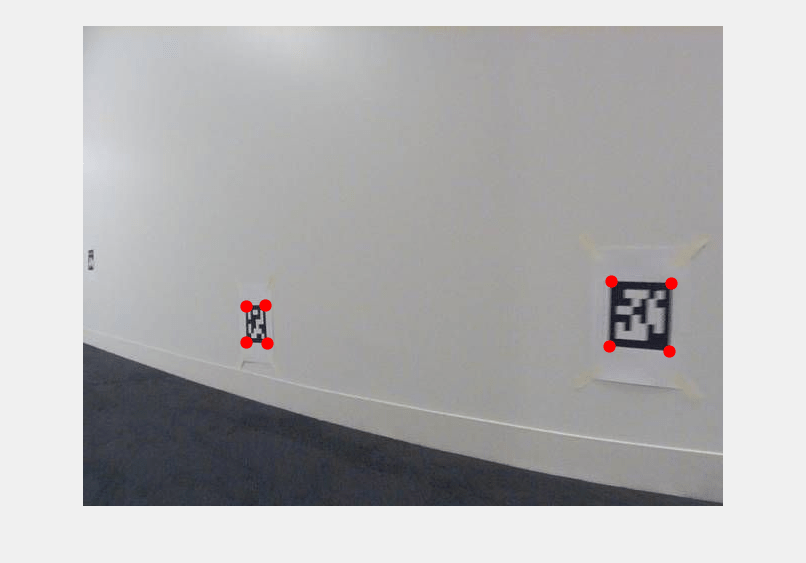Optimize Pose Graph and Inspect Results

After building the pose graph, optimize it using the optimizePoseGraph function.

pgUpd = optimizePoseGraph(pg);

Visualize both the initial and optimized pose graph. The optimized pose graph shows these improvements:

• The initial position of the robot relative to the landmarks has been corrected.

• The landmarks on each wall are better aligned.

• The vertical drift in the robot trajectory along the z direction has been corrected.

figure('Visible','on');
show(pg); axis equal; xlim([-10.0 5.0]); ylim([-2.0 12.0]);
title('Before Optimization XY View')figure('Visible','on');
show(pgUpd); axis equal; xlim([-10.0 5.0]); ylim([-2.0 12.0]);
title('After Optimization XY View')% Vertical drift.
figure('Visible','on');
subplot(2,1,1)
show(pg); xlim([-10.0 5.0]); view(0, 0)
title('Before Optimization XZ View')
subplot(2,1,2)
show(pgUpd); xlim([-10.0 5.0]); view(0, 0)
title('After Optimization XZ View')Conclusion

The final robot pose graph is overlaid on a blue print of the office area to show the true trajectory and the estimated landmark locations. If you playback the images again, you can see there is good correlation.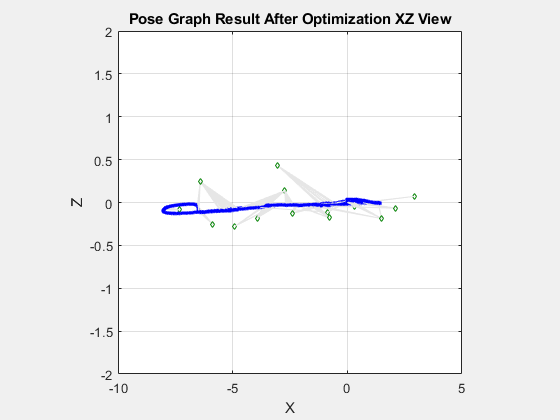Note there is one AprilTag that is not picked up by the camera, marked by red circle in the following images. These images show the position of the AprilTags for your reference.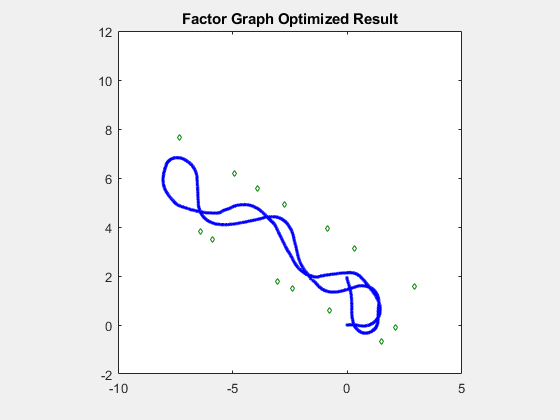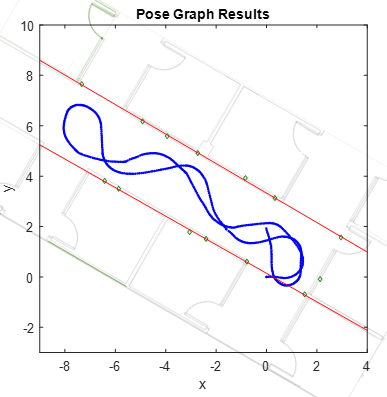Extract Sensor Data from rosbag

The exampleHelperExtractDataFromRosbag function extracts sychronized image and odometry data from a rosbag recorded on a Jackal UGV robot (apriltag_odometry_slam_dataset.bag located in the same folder as the .mat file). Import from a rosbag (ROS Toolbox) requires ROS Toolbox.

function [imageData, odomData] = exampleHelperExtractDataFromRosbag(bagName)
%exampleHelperExtractDataFromRosbag Extract synchronized image and odometry
%   data from a rosbag recorded on a Jackal UGV robot. ROS Toolbox license
%   is required to use this function.

%   Copyright 2020 The MathWorks, Inc.

bagSel = rosbag(bagName);
imgSel = bagSel.select('Topic','axis/image_raw_out/compressed'); % Image topic

% Needed for computing change between SE(2) poses
ss = stateSpaceSE2;
ss.WeightTheta = 1;

lastPoseSE2 = [];
lastT = [];

imageData = {};
odomData = {};

% Grab all the image messages
for i = 1:numel(imgObjs)
% Odom data is extracted from tf
if bagSel.canTransform('odom', 'laser', imgObjs{i}.Header.Stamp) % From odom frame to laser frame
% ith odom reading is extracted at the time of the ith image
[T, poseSE2] = translateTransformStampedMsg(Tstamped);

if isempty(lastT)
takeThisImage = true;
else
takeThisImage = true;
% Only accept a new pair of sensor measurements if the robot odom pose
% has changed more than this threshold since last accepted one
% Here we use the stateSpaceSE2's distance function to calculate the 2D pose difference
if ss.distance(poseSE2, lastPoseSE2) < 0.06
takeThisImage = false;
end
end

if takeThisImage
imageData{end+1} = I;
odomData{end+1} = T;
lastPoseSE2 = poseSE2;
lastT = T;
end
end
end

end

function [ToutSE3, poseSE2] = translateTransformStampedMsg(Tin)
%translateTransformMsg Extract the 4x4 homogeneous transformation matrix,
%   ToutSE3, from a TransformStamped message object. This function also
%   returns a second output, poseSE2, which is an SE(2) pose vector
%   computed by projecting ToutSE2 to the XY plane. Note the formulation
%   used is approximate and relies on the assumption that the robot mostly
%   moves on the flat ground.

%   Copyright 2020 The MathWorks, Inc.

%Tin - TransformStamped message object
x = Tin.Transform.Translation.X;
y = Tin.Transform.Translation.Y;
z = Tin.Transform.Translation.Z;

qx = Tin.Transform.Rotation.X;
qy = Tin.Transform.Rotation.Y;
qz = Tin.Transform.Rotation.Z;
qw = Tin.Transform.Rotation.W;
q = [ qw, qx, qy, qz]; % Note the sequence for quaternion in MATLAB

ToutSE3 = robotics.core.internal.SEHelpers.poseToTformSE3([x,y,z,q]);
YPR = quat2eul(q);
poseSE2 = [x,y,YPR(1)];
end